# Part reflection part refraction

Again, you don't need this in an exam – it is just interesting to know.

When a ray of light travels from a fast to a slow medium, for instance air to water, at different angles of incidence (degrees to the normal) you will always get some surface boundary reflection and you will always get some refraction. It is just in different proportions depending on the angle.

(Please note: In nearly all questions in exams at basic level, the proportion of light that is reflected is not taken into account)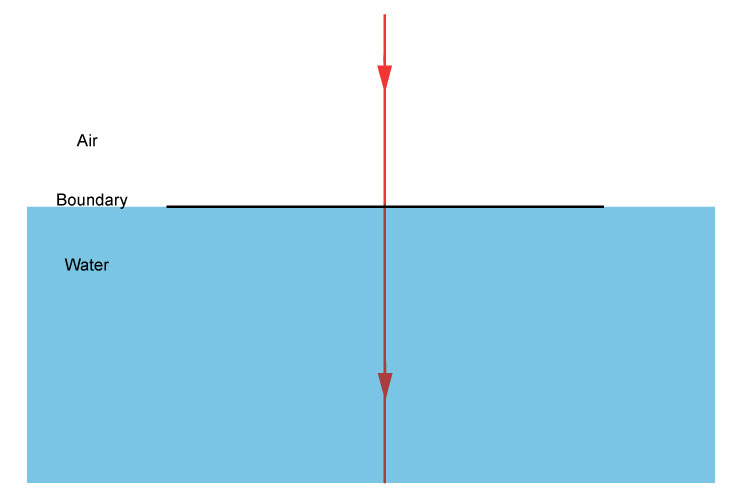Bright beam at 0° – perfectly in line with the normal line.

(3% of the light will be reflected backwards)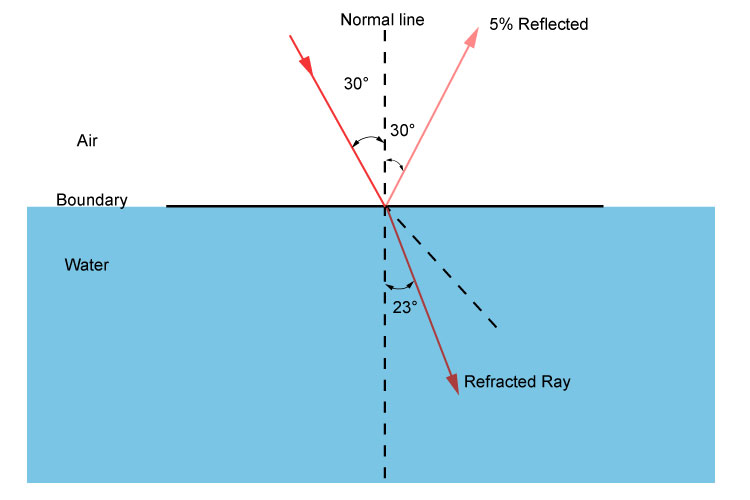Light ray in = 30^circ To the normal Reflected ray = -30^circ To the normal Dim reflection (5%) Refracted ray = 23^circ To the normal = Bright refraction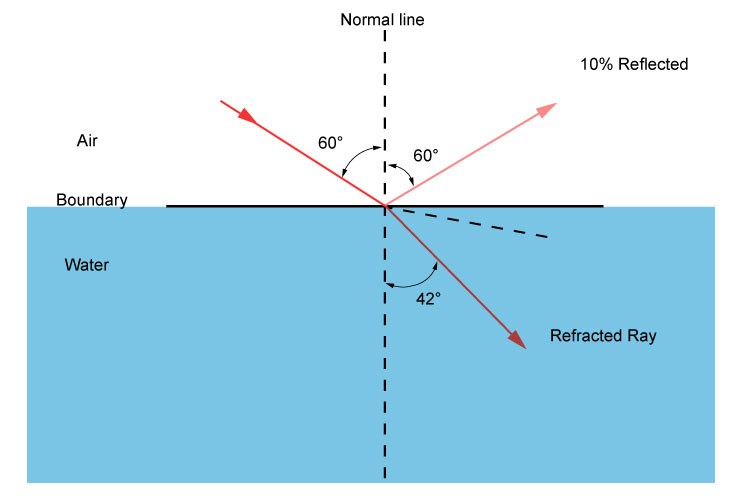Light ray in = 60^circ To the normal Reflected ray = -60^circ To the normal Dim reflection (10%) Refracted ray = 42^circ To the normal = Bright refraction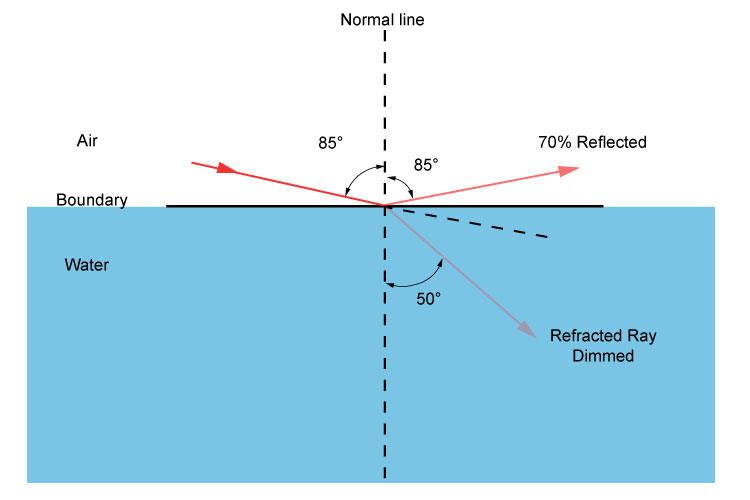Light ray in = 85^circ To the normal Reflected ray = -85^circ To the normal Bright reflection (70%) Refracted ray = 50^circ To the normal = Dim refraction

If you were to draw the amount of light refracted at the boundary when light travels from air to water you would get the following graph: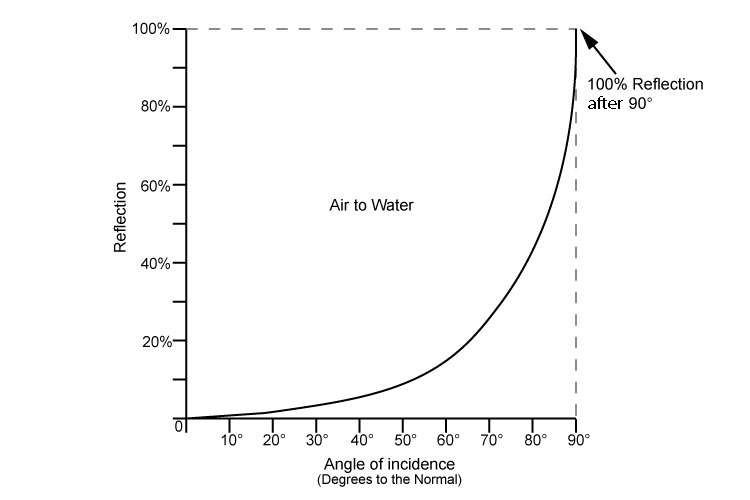Light travelling from air to water.

Light travelling at a water surface at angles of incidence less than 50 degrees mostly penetrate the water. Above 50 degrees the percentage of light that is reflected increases steeply.

So if you stand at the edge of a pool and look down near your feet (i.e. steep angle of incidence) you will see the bottom of the pool.

As you look out across the pool (i.e. at a shallow angle of incidence) you will clearly see the reflection of the surroundings and sky, and little or no detail of the bottom of the pool.